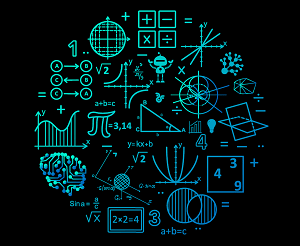# Model vs Algorithm in ML

By Learnbay Published in Artificial Intelligence 5-6 mins

## Model vs Algorithm in ML: Introduction

Machine Learning works with “models” and “algorithms”, and both play an important role in Machine Learning where the algorithm tells about the process and model is built by following those rules. So, let’s study further how Model vs Algorithm in ML( Machine Learning).

Algorithms have derived by the statistician or mathematician very long ago and those algorithms are studies and applied by the individuals for their business purposes.

A model in Machine Learning nothing but a function that is used to take some certain input, perform a certain operation which is told by algorithms to its best on the given input, and gives a suitable output.

Some of the Machine Learning algorithms are:

1. Linear regression
2. Logistic regression
3. Decision tree
4. Random forest
5. K-nearest neighbor
6. K-means learning

## What is an algorithm in Machine Learning?

An algorithm is a step by step approach powered by statistics that guides the Machine Learning in its learning process. An algorithm is nothing but one of the several components that constitute a model.

There are several characteristics of Machine Learning algorithms:

1. Machine Learning algorithms can be represented by the use of mathematics and pseudo code.
2. The effectiveness of Machine Learning algorithms can be measured and represented.
3. With any of the popular programming languages, Machine Learning algorithms can be implemented.

## What is the Model in Machine Learning?

The model is dependent on factors such as features selection, tuning parameters, cost functions along with the algorithm the model just not fully dependent on algorithms.

Model is the result of an algorithm when we implement the algorithm with the code when we train the algorithms with the real data. A model is something that tells what your program learned from the data by following the rules of those algorithms. The model is used to predict the future result that is observed by the algorithm implementation of small data.

Model = Data + Algorithm

A model contains four major steps that are:

1. Data preprocessing
2. Feature engineering
3. Data management
4. performance measurement.

## How the model and algorithms work together in Machine Learning?

For example:

y = mx+c is an equation for a line where m is the slope of the line and c is the y-intercept, this is nothing but linear regression with only one variable.

similarly, the decision tree and random forest have something like the Gini index and K-nearest having Euclidean distance formula.

So take the linear regression algorithm:

2. Find out the parameters c0, c1, c2 with the random variables.
3. Find out the learning rate alpha
4. Then repeat the following updates such as c0 = co-alpha +h(x)-y and for c1, c2 also.
5. Repeat these processes till converged.

when you employing this algorithm, you are employing these exact 5 steps in your model without changing the steps, your model initiated by the algorithm and also treat all the dataset same.

If you want to apply that algorithm to the model, the model finds out the value of m and c that we don’t know, then how will you find out?

suppose you have 3 variables that are having values of x and y now your model will find the value of m1, m2, m3, and c1, c2, c3 for three variables.

The model will work with three slopes and three intercepts to find out the result of the dataset to predict the future.

The “algorithm” might be treating all the data the same but it is the “model” that actually solves the problems. An algorithm is something that you use to train the model on the data.

After building a model, a data science enthusiasts test it to get the accuracy of that model and fine-tuning to improve the results.

## Conclusion

This article may help you yo understand about the algorithm and model (Model Vs Algorithm in ML) in Machine Learning and its relationship. In summary, an algorithm is a process or a technique that we follow to get the result or to find the solution to a problem.

And a model is a computation or a formula that formed as an output of an algorithm that takes some input, so you can say that you are building a model using a given algorithm.

Learnbay provides industry accredited data science courses in Bangalore. We understand the conjugation of technology in the field of Data science hence we offer significant courses like Machine Learning, Tensor Flow, IBM Watson, Google Cloud platform, Tableau, Hadoop, time series, R, and Python. With authentic real-time industry projects. Students will be efficient by being certified by IBM. Around hundreds of students are placed in promising companies for data science roles. Choosing Learnbay you will reach the most aspiring job of present and future.

Learnbay data science course covers Data Science with Python, Artificial Intelligence with Python, Deep Learning using Tensor-Flow. These topics are covered and co-developed with IBM.

Tags

#Artificial Intelligence